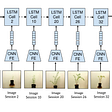# Notation of Fully Connected Neural Network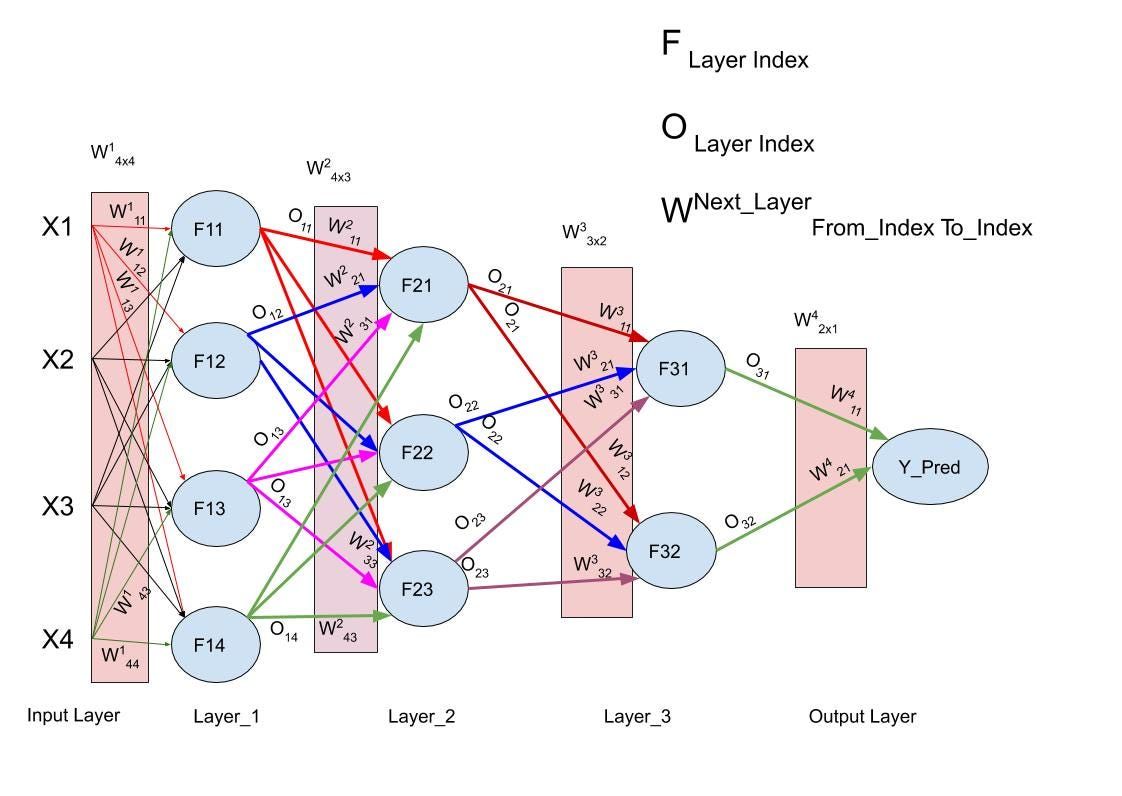This example has one Input Layer, three Hidden layers, and an Output Layer.

# Elements of Deep Neural Network

Xi is a four-dimensional feature of a data point.

Xij represents each feature of the data point.

Y_Pred is a real number. This means here we are solving a regression problem in this example.

F is the Activation Function.

O is Output.

W is the weight.

We have weights associated with each of the edges. These weights get multiply with the Inputs of the next layer and summed. This Result passes through a function F. If the input is the same as the output we call the function an Identity function. Ex. Linear Regression.

The number of weight in each layer can be represented as the “number of Input” x “the number of the output” matrix

Let's consider weights between hidden layer 1 and hidden layer 2. Layer 1 has 4 units and Layer 3 has 3 units. So, the total number of weights will be 4 * 3 = 12. Similarly, between Layer 2 and Layer 3, the number of weights will be 3 * 2 = 6.

For simplicity I excluded Bias but we can have Bias term as well.

--

--

--

## More from Chinmayi Sahu

Machine Learning Enthusiast

Love podcasts or audiobooks? Learn on the go with our new app.

## Recommended from Medium## Real Time Image Style Interpolation## Introducing TensorSpace.js — A Way to 3D Visualize Neural Networks in Browsers## Fighting Misinformation in News using NLP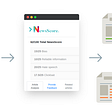## Amazon.com- Employee Access Challenge## Zero-Shot Text Classification in 5 Lines of Code## Why Deep Learning is Taking Over Machine Learning?## Convolutional Neural Network — How to code some of the critical steps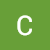## Chinmayi Sahu

Machine Learning Enthusiast

## Differentiality meets Conditional Computation## Deep Learning: Feedforward networks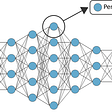## Deep introduction to LSTMs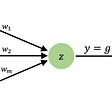## Synopsis: Intelligent Monitoring of Stress Induced by Water Deficiency in Plants Using Deep…